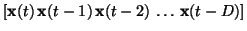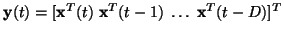Next: Results Up: Model and learning algorithm Previous: Correction of the step

## Initialisation of the factors

In the NLFA algorithm, the factors were initialised to the principal components of the observations. This could also be used in NDFA, but the drawback would be that the initialisation would only aim at factors which give a good representation for the observations but would not explicitly aim at factors which can predict the future factors and can themselves be predicted from the past factors.

Phase space embedding methods are standard techniques in the analysis of nonlinear dynamical systems and they can also be applied here. In short, the idea is that the internal state of a (deterministic) dynamical system is embedded in the sequence of observations. It may be impossible to deduce the state s(t) of the system from one measurement x(t) alone. Under suitable conditions, however, a sequenceof observations contains all the information needed to reconstruct the original state if the number D of delays is large enough .

The solution used here is to initialise the factors to principal components of a sequence of observations. In general, the state of a dynamical system is nonlinearly embedded in the sequence, but principal component analysis can nevertheless find a good starting point for the factors. To be more specific, instead of computing the principal components from x(t), they are computed from.

The NLFA algorithm could be used1 for extracting a state which is nonlinearly embedded in y(t). This would amount to computing the principal components of y(t), then using the NLFA algorithm to further refine the extracted factors and finally learning the dynamic model for x(t) starting from the factors given by the NLFA algorithm. However, here the dynamic model is included already in the second phase: the NDFA algorithm is used for finding factors which can represent the concatenated observations y(t).

The benefit of this procedure is that using the concatenated observations y(t) as observations promotes the algorithm to find factors which can represent not only the original observations x(t) but also their time behaviour. Once these factors have appeared and the dynamic mapping g(t) has adapted, the factors representing the dynamics have support from the dynamic mapping and the time lagged part of y(t) can be dropped away leaving only x(t). If the learning starts directly with x(t), there is the danger that some of the factors describing the dynamics will be effectively pruned away.Next: Results Up: Model and learning algorithm Previous: Correction of the step
Harri Valpola
2000-10-17